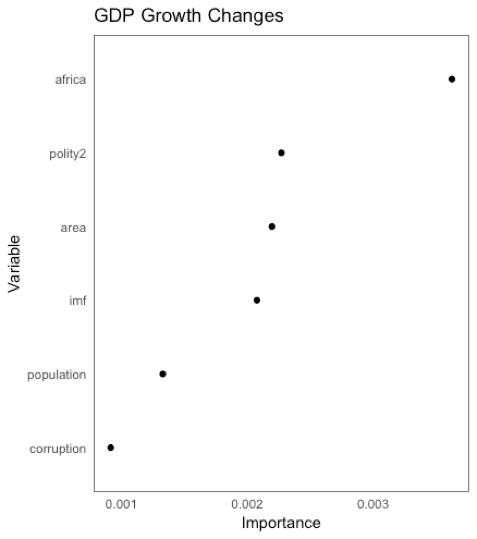# GDP Growth ChangesNote: Figure 3 presents the results from a random forest model that examines the predictors of GDP growth revisions. The vertical axis ranks variables according to their importance for predicting GDP Growth Changes. The horizontal axis displays estimates of permutation accuracy for each variable, calculated as the difference in mean squared error between a model that is fitted using the observed values for a measure and a model that is fitted using random (but realistic) values for the same measure. This measure is then scaled to represent the percentage increase in mean square error caused by permuting the values of the variable. Positive values indicate that the variables increase the predictive performance of the model, while negative values indicate that the variables decrease the predictive performance of the variables. See paper for more details.